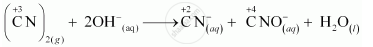CBSE (Science) Class 11CBSE
Share

# What Sorts of Informations Can You Draw from the Following Reaction ? (Cn)2(G)+2oh−(Aq)→Cn−(Aq)+Cno−(Aq)+H2ol - CBSE (Science) Class 11 - Chemistry

ConceptOxidation Number Types of Redox Reactions

#### Question

What sorts of informations can you draw from the following reaction ?

(CN)_(2(g)) + 2OH_((aq))^(-) -> CN_((aq))^(-) + CNO_((aq))^(-) + H_2O_(l)

#### Solution 1

The oxidation numbers of carbon in (CN)2, CN and CNO– are +3, +2 and +4 respectively. These are obtained as shown below:

Let the oxidation number of C be x.

(CN)2

2(x – 3) = 0

= 3

CN

x – 3 = –1

= 2

CNO

x – 3 – 2 = –1

= 4

The oxidation number of carbon in the various species is:It can be easily observed that the same compound is being reduced and oxidised simultaneously in the given equation. Reactions in which the same compound is reduced and oxidised is known as disproportionation reactions. Thus, it can be said that the alkaline decomposition of cyanogen is an example of disproportionation reaction.

#### Solution 2

Let x be the O.N. of C.
O.N. of C in cyanogen, (CN)2 = 2 (x – 3) = 0 or x = +3 O.N. of C in cyanide ion, CN- = x – 3 = -1 or x = +2 O.N. of C in cyanate ion, CNO =x-3-2 = -lora: = +4 The four information about the reaction are:

(i) The reaction involves decomposition of cyanogen, (CN)2 in the alkaline medium to cyanide ion, CN and cyanate ion,CNO.

(ii) The O.N. of C decreases from +3 in (CN)2 to +2 in CNion and increases from +3 in(CN)2 to +4 in CNO ion. Thus, cyanogen is simultaneously reduced to cyanide ion and oxidised to cyanate ion.

(iii) It is an example of a redox reaction in general and a disproportionation reaction in particular.

(iv) Cyanogen is a pseudohalogen (behaves like halogens) while cyanide ion is a pseudohalide ion (behaves like halide ion).

Is there an error in this question or solution?

#### APPEARS IN

Solution What Sorts of Informations Can You Draw from the Following Reaction ? (Cn)2(G)+2oh−(Aq)→Cn−(Aq)+Cno−(Aq)+H2ol Concept: Oxidation Number - Types of Redox Reactions.
S